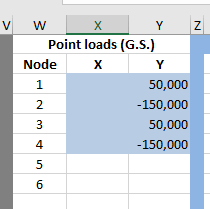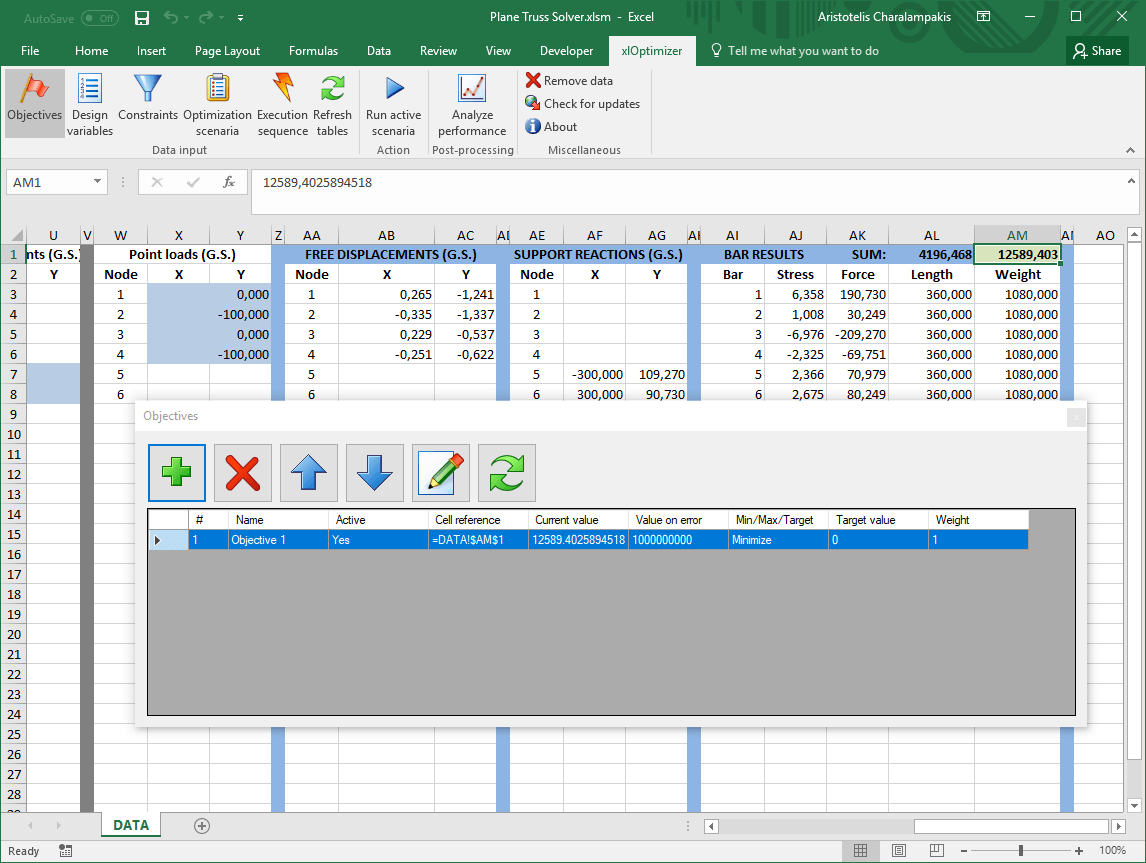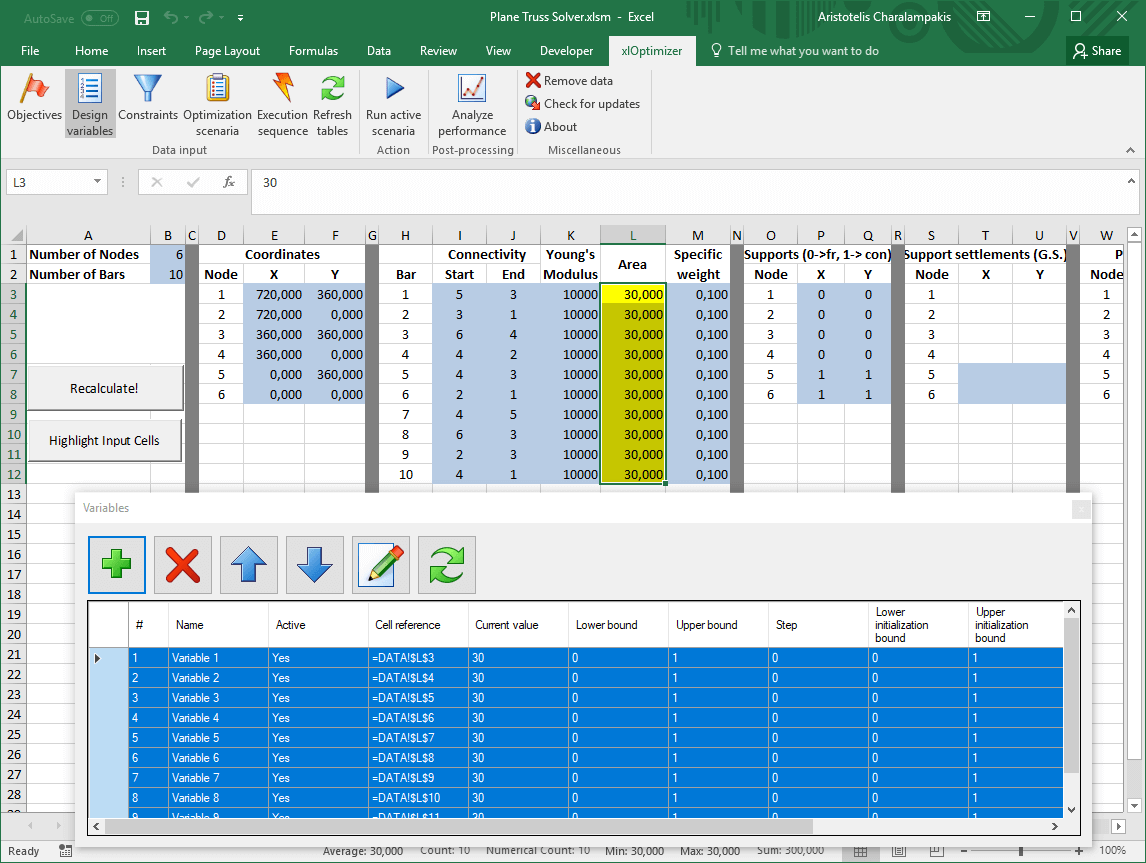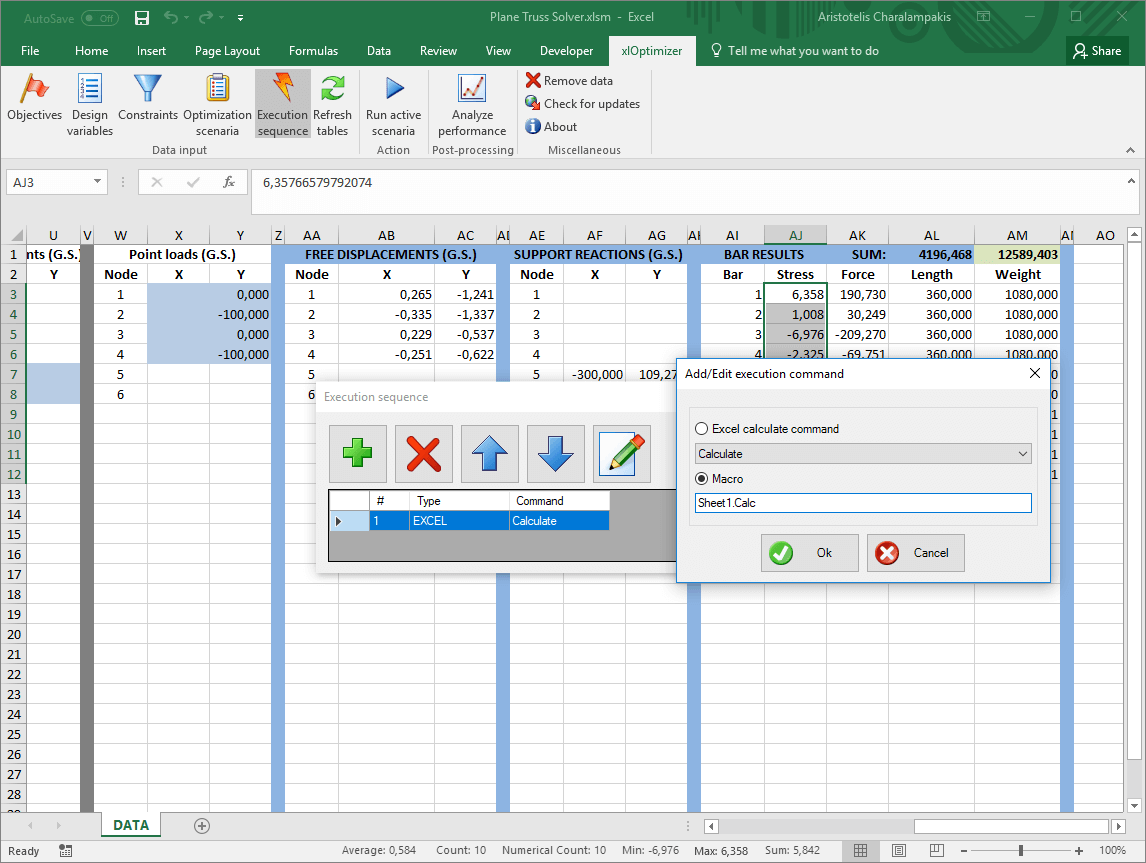## Introduction

The purpose of this example is the minimization of the weight of a planar bar truss. We will use a well-known benchmark problem, i.e. the 10-bar planar truss, which has been studied extensively in the literature. The geometrical data is given in the picture. The material's Young's modulus is E = 10000 ksi and the specific weight is ρ = 0.1 lb/in3. The purpose is to minimize its weight by picking the appropriate cross-sectional area for each bar, so that the following requirements (constraints) are met:

• The cross-sectional area of each bar varies between 0.1 and 35 in2 (these are the so-called side constraints of our design variables)
• The stress in each bar does not exceed ± 25 ksi
• The displacement in each free node in each direction should not exceed 2 inches

The 10-bar truss has two load cases. In load case 1 (LC1), P1=100 kips and P2 = 0 kips; in load case 2 (LC2), P1=150 kips and P2 = 50 kips.

This article assumes that you have read and understood the procedures described in the toy problems. These include the optimization using pure Microsoft Excel formulas and the optimization using VBA (Visual Basic for Applications).

## Step 1 : Setting-up the spreadsheet

We have already created the spreadsheet for you. It can be used for any planar truss. Note that the spreadsheet uses consistent unit system; this means that, if you pick the correct units, you can have the correct results in SI as well. In the file, however, the data has been set in the Imperial System as this was used for the definition of the 10-bar truss. Therefore, the area of each bar is given in in2 (shown in the yellow cells). The evaluation of the stresses and displacements of the truss is performed by a custom routine, i.e. Sheet1.Calc. The code can be inspected if you produce the VBA editor (by pressing Alt+F11).As mentioned above, there are two load cases. You need to change the loads manually, depending on the load case. For LC1, you need to enter the following data:Whereas for LC2, you need to enter the data as follows:## Step 2 : Setting up the objective function

Select cell AM1. This holds our objective function, i.e. the current weight of the truss. In the xlOptimizer ribbon, press Objectives to produce the list of objectives. Then press the Add button '+':The objective is set to minimization by default. Press the Objectives button in the ribbon again to hide the objectives list.

## Step 3 : Setting up the design variables

Select cells L3 to L12. These hold our design variables, i.e. the cross-sectional area of each bar. In the xlOptimizer ribbon, press Design variables to produce the list of design variables. Then press the Add button '+' to add the cells to the list:Having all design variables selected in the list, press the Edit button in the toolbar. In the form, set the bounds from 0.1 to 35 and press Ok. The selection will be applied to all selected rows.Press the Design variables button again in the ribbon to hide the design variable list.

## Step 4 : Setting up the constraints

Select cells AB3 to AC6. These hold the displacement of the free nodes. In the xlOptimizer ribbon, press Constraints to produce the list of design variables. Then press the Add button '+' to add the cells to the list. Edit the newly added rows in the list, by pressing the Edit button in the toolbar, so that the values are less than or equal to 2:However, the same cell values need to be larger than -2. Press the Add button again to add new rows (for the same cells), and edit them so that their values are larger than -2:The stresses in each bar must be between ± 25 ksi. Select cells AJ3 to AJ12, which hold the stress for each bar. Repeat the same procedure described above. In the end, 36 constraints should be included in the list:Press the Constraints button again in the ribbon to hide the constraints list.

## Step 5 : Setting up the execution sequence

In the xlOptimizer ribbon, press Execution sequence to produce the list of execution commands. By default, a single Calculate command is listed. This is a call to Microsoft Excel's internal calculation routine, which evaluates the formulas such as '=SUM(C2:C6)'. Since we do not need any such calculation (our VBA code evaluates everything, including the sums), we can substitute this command with Sheet1.Calc:Press Ok to save the changes.

## Step 6 : Setting up the optimization scenario

In the ribbon, press the Optimization scenaria button. Next, press the Add button '+' in the toolbar to add a new scenario. The following form appears:Make the appropriate selections and press Ok. The scenario is added to the list. In this case, the Standard Differential Evolution algorithm was selected. Since this algorithm uses direct comparison between solutions, and the problem includes many constraints, it is desirable to select By objective function and feasibility in the Algorithm > Comparison of solutions drop-down list. This will ensure that the best solutions will be free of any constraint violations.

The data input is now complete. In the ribbon, press the Optimization scenaria button again to hide the list.

## Step 7 : Optimization

We can now proceed to the optimization by pressing the Run active scenaria button.

The file for this example can be downloaded here. Note that it is saved a macro enabled book with the extension .xlsm. You need to enable macros to run it.

By inspection of the literature, for LC1, the best solution for the 10-bar truss that does not violate any constraints has a weight of 5060.855 lbs. For LC2, the weight is 4676.932 lbs.